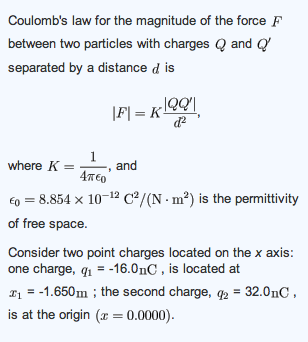# Problem: What is the net force exerted by these two charges on a third charge q3= 45.0nC placed between q1 and q2 and at x3=-1.210m?

###### FREE Expert Solution

As the question states, we use Coulomb's law to get the electric force as:

$\overline{){\mathbf{F}}{\mathbf{=}}\frac{\mathbf{k}{\mathbf{q}}_{\mathbf{1}}{\mathbf{q}}_{\mathbf{2}}}{{\mathbf{r}}^{\mathbf{2}}}}$, where F is the electric force, k is Coulomb constant, and q1 and q2 are charges separated by distance r.

The distance of separation from charge 1 to charge 3 is:

r13 = - 1.650 - (-1.210) = - 0.440 m

The electric force that q1 exerts on q3 is:

88% (264 ratings)###### Problem DetailsWhat is the net force exerted by these two charges on a third charge q3= 45.0nC placed between q1 and q2 and at x3=-1.210m?

Frequently Asked Questions

What scientific concept do you need to know in order to solve this problem?

Our tutors have indicated that to solve this problem you will need to apply the Coulomb's Law (Electric Force) concept. You can view video lessons to learn Coulomb's Law (Electric Force). Or if you need more Coulomb's Law (Electric Force) practice, you can also practice Coulomb's Law (Electric Force) practice problems.